Publicité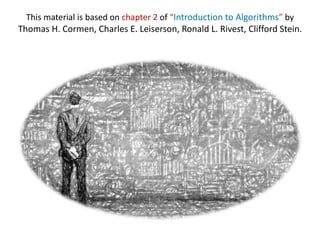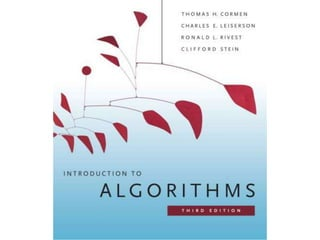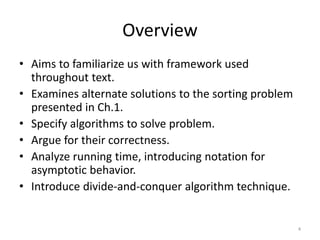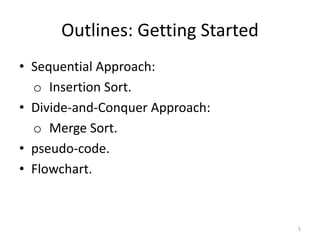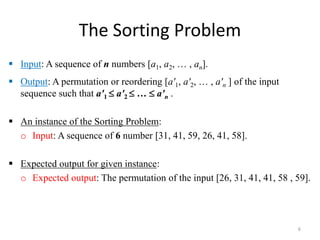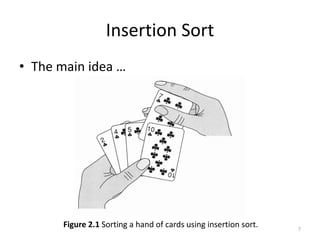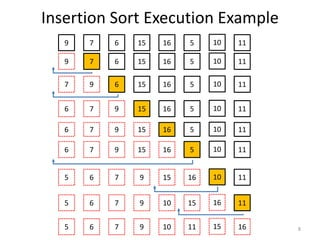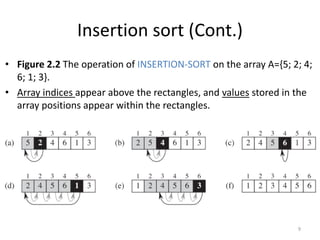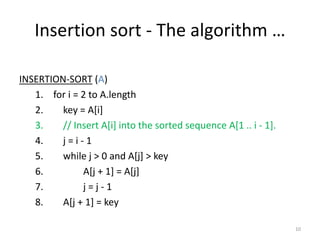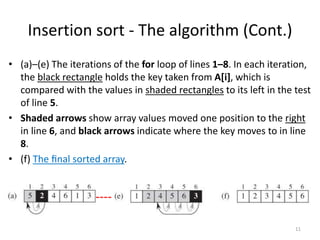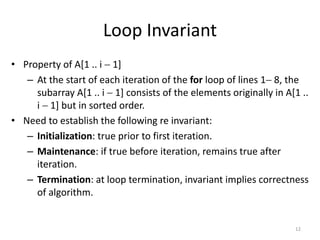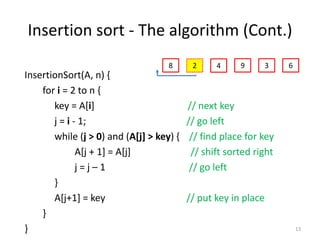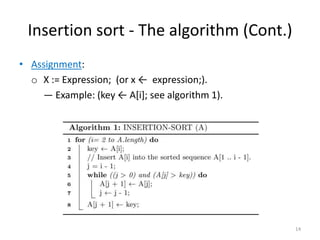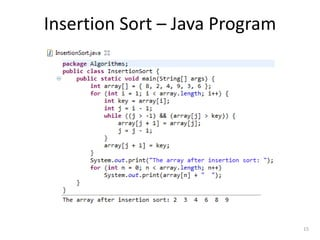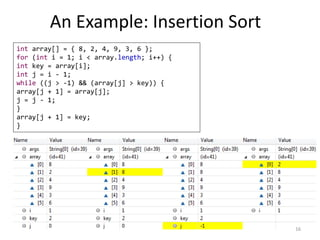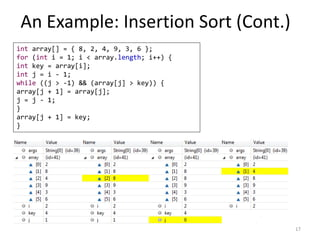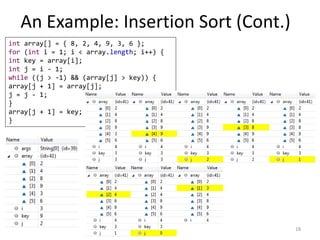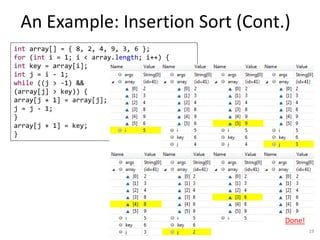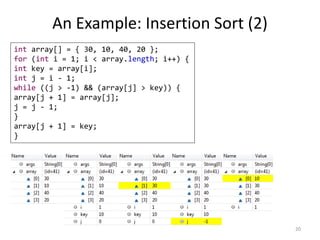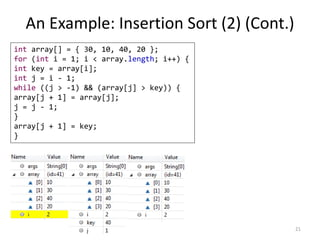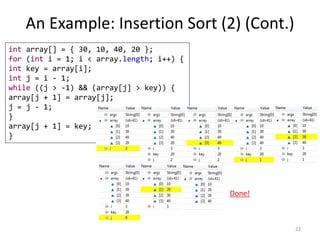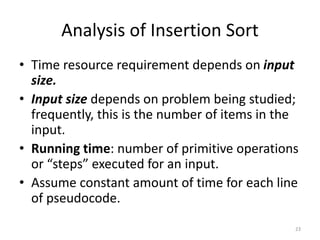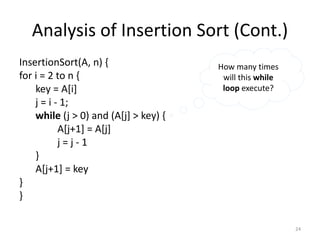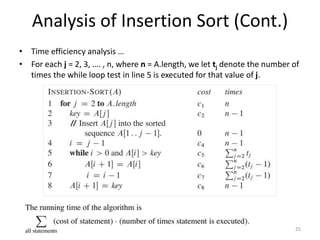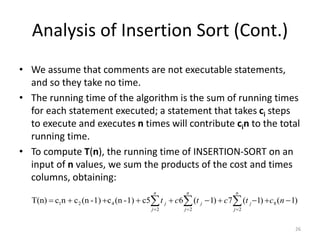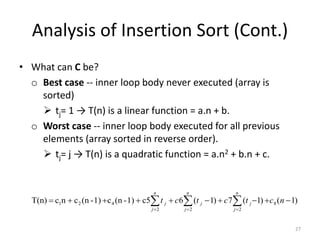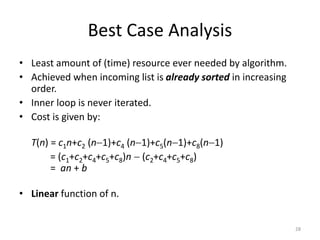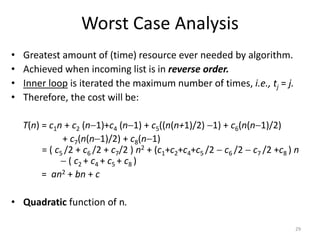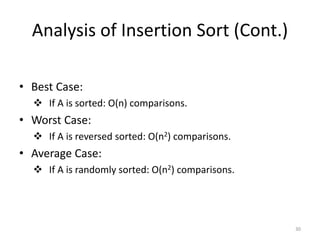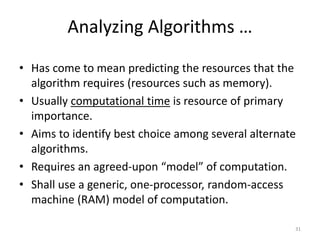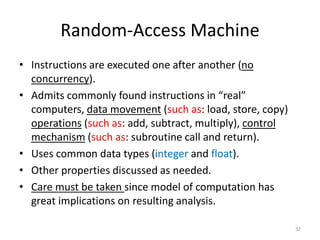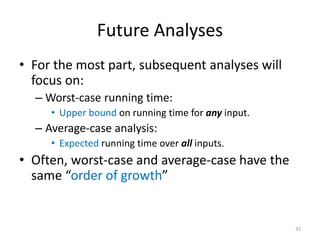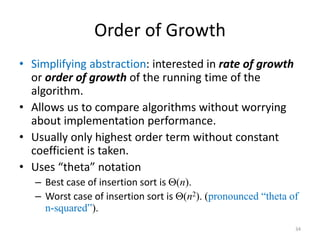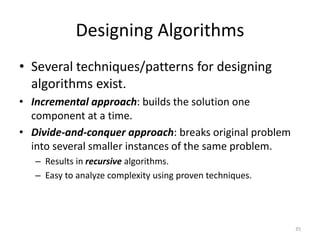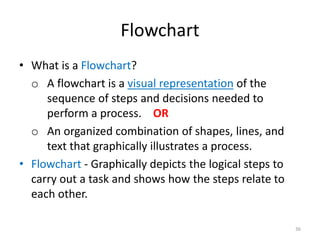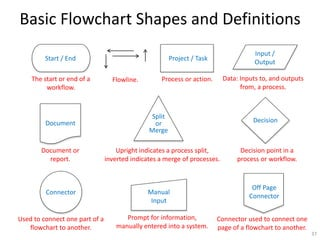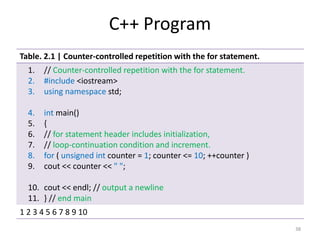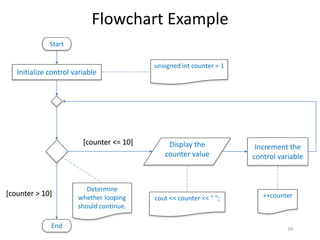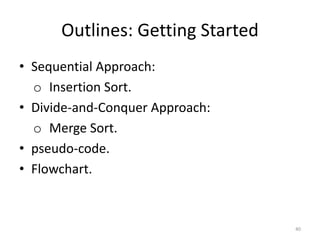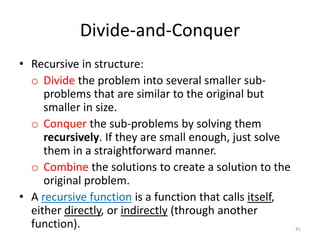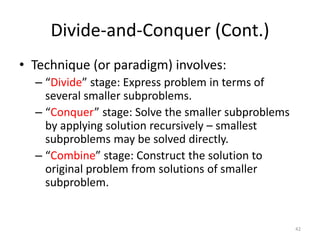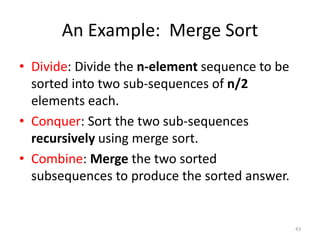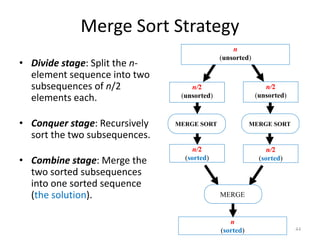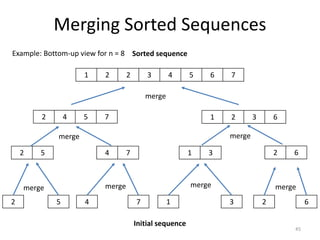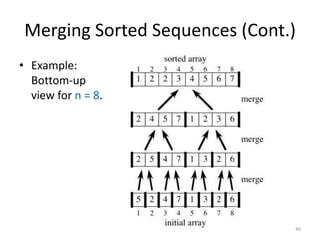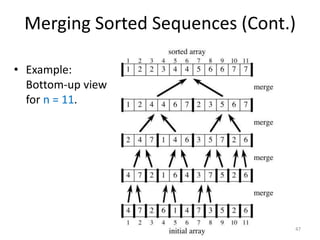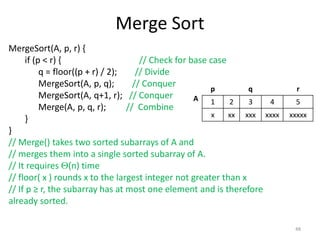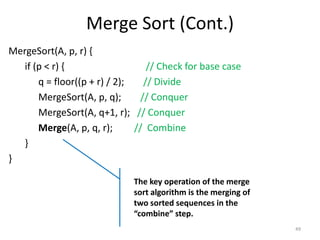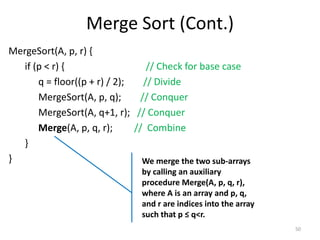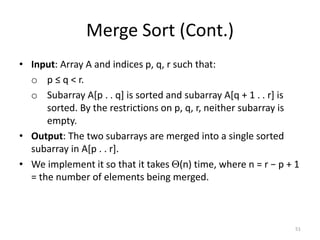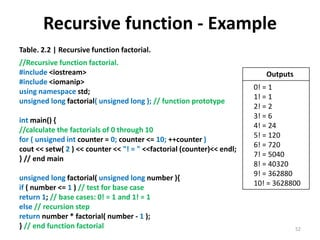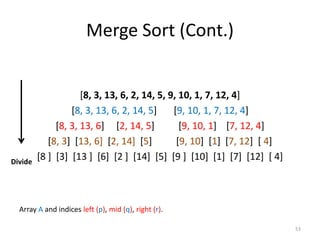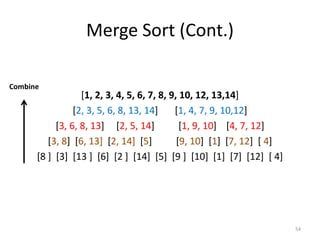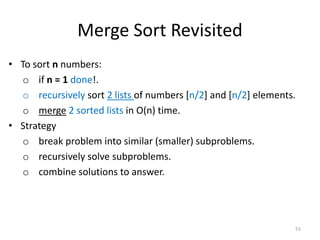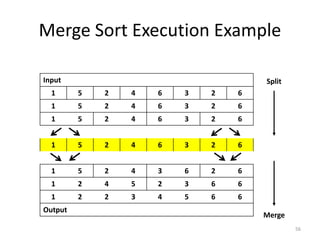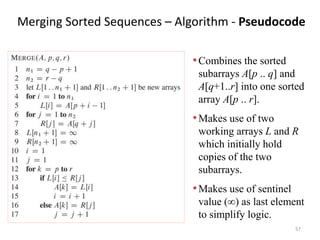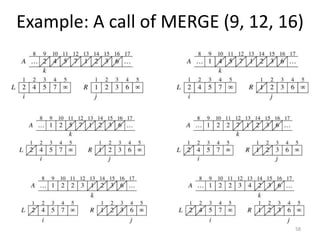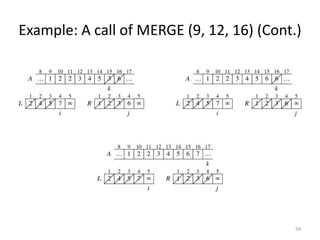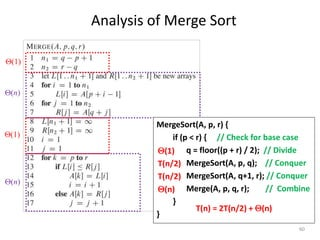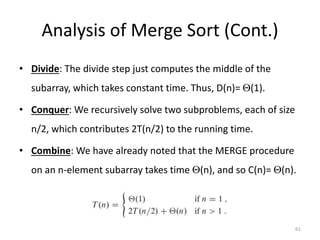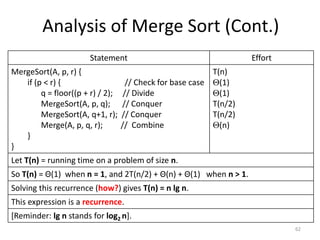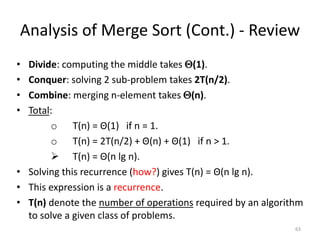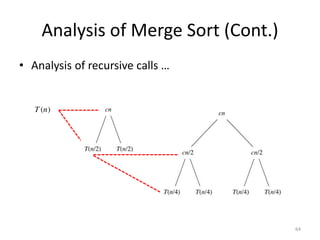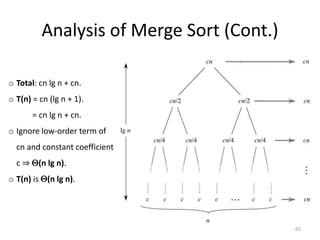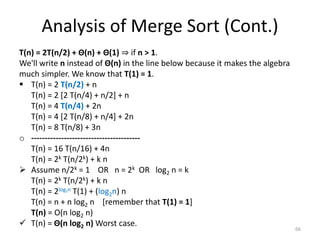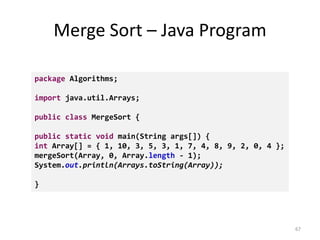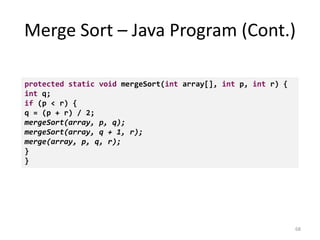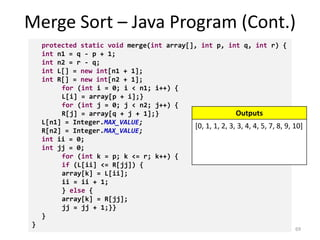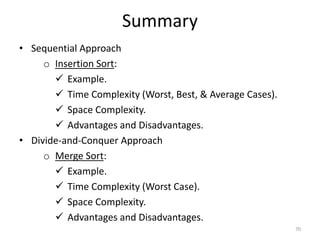1 sur 71
Publicité

### Algorithms - "Chapter 2 getting started"

1. Chapter 2: Getting Started Mutah University Faculty of IT, Department of Software Engineering Dr. Ra’Fat A. AL-msie’deen Algorithms
2. This material is based on chapter 2 of “Introduction to Algorithms” by Thomas H. Cormen, Charles E. Leiserson, Ronald L. Rivest, Clifford Stein.
3. Overview • Aims to familiarize us with framework used throughout text. • Examines alternate solutions to the sorting problem presented in Ch.1. • Specify algorithms to solve problem. • Argue for their correctness. • Analyze running time, introducing notation for asymptotic behavior. • Introduce divide-and-conquer algorithm technique. 4
4. Outlines: Getting Started • Sequential Approach: o Insertion Sort. • Divide-and-Conquer Approach: o Merge Sort. • pseudo-code. • Flowchart. 5
5. The Sorting Problem  Input: A sequence of n numbers [a1, a2, … , an].  Output: A permutation or reordering [a'1, a'2, … , a'n ] of the input sequence such that a'1  a'2  …  a'n .  An instance of the Sorting Problem: o Input: A sequence of 6 number [31, 41, 59, 26, 41, 58].  Expected output for given instance: o Expected output: The permutation of the input [26, 31, 41, 41, 58 , 59]. 6
6. Insertion Sort • The main idea … 7 Figure 2.1 Sorting a hand of cards using insertion sort.
7. Insertion Sort Execution Example 8 9 7 6 1015 16 5 11 9 6 1015 16 5 11 7 9 1015 16 5 11 6 7 9 1015 16 11 5 6 7 9 15 16 11 5 6 7 169 10 15 5 6 7 159 10 11 16 7 6 5 10 11 6 7 1015 16 5 119 6 7 1015 16 5 119
8. Insertion sort (Cont.) • Figure 2.2 The operation of INSERTION-SORT on the array A={5; 2; 4; 6; 1; 3}. • Array indices appear above the rectangles, and values stored in the array positions appear within the rectangles. 9
9. Insertion sort - The algorithm … 10 INSERTION-SORT (A) 1. for i = 2 to A.length 2. key = A[i] 3. // Insert A[i] into the sorted sequence A[1 .. i - 1]. 4. j = i - 1 5. while j > 0 and A[j] > key 6. A[j + 1] = A[j] 7. j = j - 1 8. A[j + 1] = key
10. Insertion sort - The algorithm (Cont.) • (a)–(e) The iterations of the for loop of lines 1–8. In each iteration, the black rectangle holds the key taken from A[i], which is compared with the values in shaded rectangles to its left in the test of line 5. • Shaded arrows show array values moved one position to the right in line 6, and black arrows indicate where the key moves to in line 8. • (f) The ﬁnal sorted array. 11
11. Loop Invariant • Property of A[1 .. i  1] – At the start of each iteration of the for loop of lines 1 8, the subarray A[1 .. i  1] consists of the elements originally in A[1 .. i  1] but in sorted order. • Need to establish the following re invariant: – Initialization: true prior to first iteration. – Maintenance: if true before iteration, remains true after iteration. – Termination: at loop termination, invariant implies correctness of algorithm. 12
12. Insertion sort - The algorithm (Cont.) InsertionSort(A, n) { for i = 2 to n { key = A[i] // next key j = i - 1; // go left while (j > 0) and (A[j] > key) { // find place for key A[j + 1] = A[j] // shift sorted right j = j – 1 // go left } A[j+1] = key // put key in place } } 13 8 4 9 3 62
13. Insertion sort - The algorithm (Cont.) • Assignment: o X := Expression; (or x ← expression;). ― Example: (key ← A[i]; see algorithm 1). 14
14. Insertion Sort – Java Program 15
15. An Example: Insertion Sort int array[] = { 8, 2, 4, 9, 3, 6 }; for (int i = 1; i < array.length; i++) { int key = array[i]; int j = i - 1; while ((j > -1) && (array[j] > key)) { array[j + 1] = array[j]; j = j - 1; } array[j + 1] = key; } 16
16. An Example: Insertion Sort (Cont.) int array[] = { 8, 2, 4, 9, 3, 6 }; for (int i = 1; i < array.length; i++) { int key = array[i]; int j = i - 1; while ((j > -1) && (array[j] > key)) { array[j + 1] = array[j]; j = j - 1; } array[j + 1] = key; } 17
17. An Example: Insertion Sort (Cont.) int array[] = { 8, 2, 4, 9, 3, 6 }; for (int i = 1; i < array.length; i++) { int key = array[i]; int j = i - 1; while ((j > -1) && (array[j] > key)) { array[j + 1] = array[j]; j = j - 1; } array[j + 1] = key; } 18
18. An Example: Insertion Sort (Cont.) int array[] = { 8, 2, 4, 9, 3, 6 }; for (int i = 1; i < array.length; i++) { int key = array[i]; int j = i - 1; while ((j > -1) && (array[j] > key)) { array[j + 1] = array[j]; j = j - 1; } array[j + 1] = key; } 19 Done!
19. An Example: Insertion Sort (2) 20 int array[] = { 30, 10, 40, 20 }; for (int i = 1; i < array.length; i++) { int key = array[i]; int j = i - 1; while ((j > -1) && (array[j] > key)) { array[j + 1] = array[j]; j = j - 1; } array[j + 1] = key; }
20. An Example: Insertion Sort (2) (Cont.) 21 int array[] = { 30, 10, 40, 20 }; for (int i = 1; i < array.length; i++) { int key = array[i]; int j = i - 1; while ((j > -1) && (array[j] > key)) { array[j + 1] = array[j]; j = j - 1; } array[j + 1] = key; }
21. An Example: Insertion Sort (2) (Cont.) 22 int array[] = { 30, 10, 40, 20 }; for (int i = 1; i < array.length; i++) { int key = array[i]; int j = i - 1; while ((j > -1) && (array[j] > key)) { array[j + 1] = array[j]; j = j - 1; } array[j + 1] = key; } Done!
22. Analysis of Insertion Sort • Time resource requirement depends on input size. • Input size depends on problem being studied; frequently, this is the number of items in the input. • Running time: number of primitive operations or “steps” executed for an input. • Assume constant amount of time for each line of pseudocode. 23
23. Analysis of Insertion Sort (Cont.) InsertionSort(A, n) { for i = 2 to n { key = A[i] j = i - 1; while (j > 0) and (A[j] > key) { A[j+1] = A[j] j = j - 1 } A[j+1] = key } } 24 How many times will this while loop execute?
24. Analysis of Insertion Sort (Cont.) • Time efficiency analysis … • For each j = 2, 3, …. , n, where n = A.length, we let tj denote the number of times the while loop test in line 5 is executed for that value of j. 25
25. Analysis of Insertion Sort (Cont.) • We assume that comments are not executable statements, and so they take no time. • The running time of the algorithm is the sum of running times for each statement executed; a statement that takes ci steps to execute and executes n times will contribute cin to the total running time. • To compute T(n), the running time of INSERTION-SORT on an input of n values, we sum the products of the cost and times columns, obtaining: 26    n j j n j j n j j nctctct 2 8 22 421 )1()1(7)1(6c51)-(nc1)-(ncncT(n)
26. Analysis of Insertion Sort (Cont.) • What can C be? o Best case -- inner loop body never executed (array is sorted)  tj= 1 → T(n) is a linear function = a.n + b. o Worst case -- inner loop body executed for all previous elements (array sorted in reverse order).  tj= j → T(n) is a quadratic function = a.n2 + b.n + c. 27    n j j n j j n j j nctctct 2 8 22 421 )1()1(7)1(6c51)-(nc1)-(ncncT(n)
27. Best Case Analysis • Least amount of (time) resource ever needed by algorithm. • Achieved when incoming list is already sorted in increasing order. • Inner loop is never iterated. • Cost is given by: T(n) = c1n+c2 (n1)+c4 (n1)+c5(n1)+c8(n1) = (c1+c2+c4+c5+c8)n  (c2+c4+c5+c8) = an + b • Linear function of n. 28
28. Worst Case Analysis • Greatest amount of (time) resource ever needed by algorithm. • Achieved when incoming list is in reverse order. • Inner loop is iterated the maximum number of times, i.e., tj = j. • Therefore, the cost will be: T(n) = c1n + c2 (n1)+c4 (n1) + c5((n(n+1)/2) 1) + c6(n(n1)/2) + c7(n(n1)/2) + c8(n1) = ( c5 /2 + c6 /2 + c7/2 ) n2 + (c1+c2+c4+c5 /2  c6 /2  c7 /2 +c8 ) n  ( c2 + c4 + c5 + c8 ) = an2 + bn + c • Quadratic function of n. 29
29. Analysis of Insertion Sort (Cont.) • Best Case:  If A is sorted: O(n) comparisons. • Worst Case:  If A is reversed sorted: O(n2) comparisons. • Average Case:  If A is randomly sorted: O(n2) comparisons. 30
30. Analyzing Algorithms … • Has come to mean predicting the resources that the algorithm requires (resources such as memory). • Usually computational time is resource of primary importance. • Aims to identify best choice among several alternate algorithms. • Requires an agreed-upon “model” of computation. • Shall use a generic, one-processor, random-access machine (RAM) model of computation. 31
31. Random-Access Machine • Instructions are executed one after another (no concurrency). • Admits commonly found instructions in “real” computers, data movement (such as: load, store, copy) operations (such as: add, subtract, multiply), control mechanism (such as: subroutine call and return). • Uses common data types (integer and float). • Other properties discussed as needed. • Care must be taken since model of computation has great implications on resulting analysis. 32
32. Future Analyses • For the most part, subsequent analyses will focus on: – Worst-case running time: • Upper bound on running time for any input. – Average-case analysis: • Expected running time over all inputs. • Often, worst-case and average-case have the same “order of growth” 33
33. Order of Growth • Simplifying abstraction: interested in rate of growth or order of growth of the running time of the algorithm. • Allows us to compare algorithms without worrying about implementation performance. • Usually only highest order term without constant coefficient is taken. • Uses “theta” notation – Best case of insertion sort is (n). – Worst case of insertion sort is (n2). (pronounced “theta of n-squared”). 34
34. Designing Algorithms • Several techniques/patterns for designing algorithms exist. • Incremental approach: builds the solution one component at a time. • Divide-and-conquer approach: breaks original problem into several smaller instances of the same problem. – Results in recursive algorithms. – Easy to analyze complexity using proven techniques. 35
35. Flowchart • What is a Flowchart? o A flowchart is a visual representation of the sequence of steps and decisions needed to perform a process. OR o An organized combination of shapes, lines, and text that graphically illustrates a process. • Flowchart - Graphically depicts the logical steps to carry out a task and shows how the steps relate to each other. 36
36. Basic Flowchart Shapes and Definitions 37 Start / End The start or end of a workflow. Project / Task Process or action. Split or Merge Upright indicates a process split, inverted indicates a merge of processes. Off Page Connector Connector used to connect one page of a flowchart to another. Connector Used to connect one part of a flowchart to another. Decision Decision point in a process or workflow. Input / Output Data: Inputs to, and outputs from, a process. Document Document or report. Manual Input Prompt for information, manually entered into a system. Flowline.
37. C++ Program 38 Table. 2.1 | Counter-controlled repetition with the for statement. 1. // Counter-controlled repetition with the for statement. 2. #include <iostream> 3. using namespace std; 4. int main() 5. { 6. // for statement header includes initialization, 7. // loop-continuation condition and increment. 8. for ( unsigned int counter = 1; counter <= 10; ++counter ) 9. cout << counter << " "; 10. cout << endl; // output a newline 11. } // end main 1 2 3 4 5 6 7 8 9 10
38. Flowchart Example 39End Initialize control variable Increment the control variable Display the counter value [counter <= 10] [counter > 10] Start unsigned int counter = 1 ++countercout << counter << " "; Determine whether looping should continue.
39. Outlines: Getting Started • Sequential Approach: o Insertion Sort. • Divide-and-Conquer Approach: o Merge Sort. • pseudo-code. • Flowchart. 40
40. Divide-and-Conquer • Recursive in structure: o Divide the problem into several smaller sub- problems that are similar to the original but smaller in size. o Conquer the sub-problems by solving them recursively. If they are small enough, just solve them in a straightforward manner. o Combine the solutions to create a solution to the original problem. • A recursive function is a function that calls itself, either directly, or indirectly (through another function). 41
41. Divide-and-Conquer (Cont.) • Technique (or paradigm) involves: – “Divide” stage: Express problem in terms of several smaller subproblems. – “Conquer” stage: Solve the smaller subproblems by applying solution recursively – smallest subproblems may be solved directly. – “Combine” stage: Construct the solution to original problem from solutions of smaller subproblem. 42
42. An Example: Merge Sort • Divide: Divide the n-element sequence to be sorted into two sub-sequences of n/2 elements each. • Conquer: Sort the two sub-sequences recursively using merge sort. • Combine: Merge the two sorted subsequences to produce the sorted answer. 43
43. Merge Sort Strategy • Divide stage: Split the n- element sequence into two subsequences of n/2 elements each. • Conquer stage: Recursively sort the two subsequences. • Combine stage: Merge the two sorted subsequences into one sorted sequence (the solution). 44 n (sorted) MERGE n (unsorted) n/2 (unsorted) n/2 (unsorted) MERGE SORT MERGE SORT n/2 (sorted) n/2 (sorted)
44. Merging Sorted Sequences 1 2 2 3 4 5 6 7 45 Sorted sequence 2 4 5 7 1 2 3 6 2 5 4 7 1 3 2 6 2 5 4 7 1 3 2 6 merge merge merge Initial sequence mergemergemergemerge Example: Bottom-up view for n = 8
45. Merging Sorted Sequences (Cont.) • Example: Bottom-up view for n = 8. 46
46. Merging Sorted Sequences (Cont.) • Example: Bottom-up view for n = 11. 47
47. Merge Sort MergeSort(A, p, r) { if (p < r) { // Check for base case q = floor((p + r) / 2); // Divide MergeSort(A, p, q); // Conquer MergeSort(A, q+1, r); // Conquer Merge(A, p, q, r); // Combine } } // Merge() takes two sorted subarrays of A and // merges them into a single sorted subarray of A. // It requires (n) time // floor( x ) rounds x to the largest integer not greater than x // If p ≥ r, the subarray has at most one element and is therefore already sorted. 48 A p q r 1 2 3 4 5 x xx xxx xxxx xxxxx
48. Merge Sort (Cont.) MergeSort(A, p, r) { if (p < r) { // Check for base case q = floor((p + r) / 2); // Divide MergeSort(A, p, q); // Conquer MergeSort(A, q+1, r); // Conquer Merge(A, p, q, r); // Combine } } 49 The key operation of the merge sort algorithm is the merging of two sorted sequences in the “combine” step.
49. Merge Sort (Cont.) MergeSort(A, p, r) { if (p < r) { // Check for base case q = floor((p + r) / 2); // Divide MergeSort(A, p, q); // Conquer MergeSort(A, q+1, r); // Conquer Merge(A, p, q, r); // Combine } } 50 We merge the two sub-arrays by calling an auxiliary procedure Merge(A, p, q, r), where A is an array and p, q, and r are indices into the array such that p ≤ q<r.
50. Merge Sort (Cont.) • Input: Array A and indices p, q, r such that: o p ≤ q < r. o Subarray A[p . . q] is sorted and subarray A[q + 1 . . r] is sorted. By the restrictions on p, q, r, neither subarray is empty. • Output: The two subarrays are merged into a single sorted subarray in A[p . . r]. • We implement it so that it takes (n) time, where n = r − p + 1 = the number of elements being merged. 51
51. Recursive function - Example Table. 2.2 | Recursive function factorial. //Recursive function factorial. #include <iostream> #include <iomanip> using namespace std; unsigned long factorial( unsigned long ); // function prototype int main() { //calculate the factorials of 0 through 10 for ( unsigned int counter = 0; counter <= 10; ++counter ) cout << setw( 2 ) << counter << "! = " <<factorial (counter)<< endl; } // end main unsigned long factorial( unsigned long number ){ if ( number <= 1 ) // test for base case return 1; // base cases: 0! = 1 and 1! = 1 else // recursion step return number * factorial( number - 1 ); } // end function factorial 52 Outputs 0! = 1 1! = 1 2! = 2 3! = 6 4! = 24 5! = 120 6! = 720 7! = 5040 8! = 40320 9! = 362880 10! = 3628800
52. Merge Sort (Cont.) 53 [8, 3, 13, 6, 2, 14, 5, 9, 10, 1, 7, 12, 4] [8, 3, 13, 6, 2, 14, 5] [9, 10, 1, 7, 12, 4] [8, 3, 13, 6] [2, 14, 5] [9, 10, 1] [7, 12, 4] [8, 3] [13, 6] [2, 14]  [9, 10]  [7, 12] [ 4] [8 ]  [13 ]  [2 ]   [9 ]     [ 4]Divide Array A and indices left (p), mid (q), right (r).
53. Merge Sort (Cont.) 54 [1, 2, 3, 4, 5, 6, 7, 8, 9, 10, 12, 13,14] [2, 3, 5, 6, 8, 13, 14] [1, 4, 7, 9, 10,12] [3, 6, 8, 13] [2, 5, 14] [1, 9, 10] [4, 7, 12] [3, 8] [6, 13] [2, 14]  [9, 10]  [7, 12] [ 4] [8 ]  [13 ]  [2 ]   [9 ]     [ 4] Combine
54. Merge Sort Revisited • To sort n numbers: o if n = 1 done!. o recursively sort 2 lists of numbers [n/2] and [n/2] elements. o merge 2 sorted lists in O(n) time. • Strategy o break problem into similar (smaller) subproblems. o recursively solve subproblems. o combine solutions to answer. 55
55. Merge Sort Execution Example 56 Input 1 5 2 4 6 3 2 6 1 5 2 4 6 3 2 6 1 5 2 4 6 3 2 6 1 5 2 4 6 3 2 6 1 5 2 4 3 6 2 6 1 2 4 5 2 3 6 6 1 2 2 3 4 5 6 6 Output Split Merge
56. Merging Sorted Sequences – Algorithm - Pseudocode 57 •Combines the sorted subarrays A[p .. q] and A[q+1..r] into one sorted array A[p .. r]. •Makes use of two working arrays L and R which initially hold copies of the two subarrays. •Makes use of sentinel value () as last element to simplify logic.
57. Example: A call of MERGE (9, 12, 16) 58
58. Example: A call of MERGE (9, 12, 16) (Cont.) 59
59. Analysis of Merge Sort 60 MergeSort(A, p, r) { if (p < r) { // Check for base case q = floor((p + r) / 2); // Divide MergeSort(A, p, q); // Conquer MergeSort(A, q+1, r); // Conquer Merge(A, p, q, r); // Combine } } (1) (n) (n) (1) (1) T(n/2) T(n/2) (n) T(n) = 2T(n/2) + (n)
60. Analysis of Merge Sort (Cont.) • Divide: The divide step just computes the middle of the subarray, which takes constant time. Thus, D(n)= (1). • Conquer: We recursively solve two subproblems, each of size n/2, which contributes 2T(n/2) to the running time. • Combine: We have already noted that the MERGE procedure on an n-element subarray takes time (n), and so C(n)= (n). 61
61. Analysis of Merge Sort (Cont.) Statement Effort MergeSort(A, p, r) { if (p < r) { // Check for base case q = floor((p + r) / 2); // Divide MergeSort(A, p, q); // Conquer MergeSort(A, q+1, r); // Conquer Merge(A, p, q, r); // Combine } } T(n) (1) (1) T(n/2) T(n/2) (n) Let T(n) = running time on a problem of size n. So T(n) = Θ(1) when n = 1, and 2T(n/2) + Θ(n) + Θ(1) when n > 1. Solving this recurrence (how?) gives T(n) = n lg n. This expression is a recurrence. [Reminder: lg n stands for log2 n]. 62
62. Analysis of Merge Sort (Cont.) - Review • Divide: computing the middle takes (1). • Conquer: solving 2 sub-problem takes 2T(n/2). • Combine: merging n-element takes (n). • Total: o T(n) = Θ(1) if n = 1. o T(n) = 2T(n/2) + Θ(n) + Θ(1) if n > 1.  T(n) = Θ(n lg n). • Solving this recurrence (how?) gives T(n) = Θ(n lg n). • This expression is a recurrence. • T(n) denote the number of operations required by an algorithm to solve a given class of problems. 63
63. Analysis of Merge Sort (Cont.) • Analysis of recursive calls … 64
64. Analysis of Merge Sort (Cont.) 65 o Total: cn lg n + cn. o T(n) = cn (lg n + 1). = cn lg n + cn. o Ignore low-order term of cn and constant coefficient c ⇒ (n lg n). o T(n) is (n lg n).
65. T(n) = 2T(n/2) + Θ(n) + Θ(1) ⇒ if n > 1. We'll write n instead of Θ(n) in the line below because it makes the algebra much simpler. We know that T(1) = 1.  T(n) = 2 T(n/2) + n T(n) = 2 [2 T(n/4) + n/2] + n T(n) = 4 T(n/4) + 2n T(n) = 4 [2 T(n/8) + n/4] + 2n T(n) = 8 T(n/8) + 3n o ---------------------------------------- T(n) = 16 T(n/16) + 4n T(n) = 2k T(n/2k) + k n  Assume n/2k = 1 OR n = 2k OR log2 n = k T(n) = 2k T(n/2k) + k n T(n) = 2log2n T(1) + (log2n) n T(n) = n + n log2 n [remember that T(1) = 1] T(n) = O(n log2 n)  T(n) = Θ(n log2 n) Worst case. 66 Analysis of Merge Sort (Cont.)
66. Merge Sort – Java Program 67 package Algorithms; import java.util.Arrays; public class MergeSort { public static void main(String args[]) { int Array[] = { 1, 10, 3, 5, 3, 1, 7, 4, 8, 9, 2, 0, 4 }; mergeSort(Array, 0, Array.length - 1); System.out.println(Arrays.toString(Array)); }
67. Merge Sort – Java Program (Cont.) 68 protected static void mergeSort(int array[], int p, int r) { int q; if (p < r) { q = (p + r) / 2; mergeSort(array, p, q); mergeSort(array, q + 1, r); merge(array, p, q, r); } }
68. Merge Sort – Java Program (Cont.) 69 protected static void merge(int array[], int p, int q, int r) { int n1 = q - p + 1; int n2 = r - q; int L[] = new int[n1 + 1]; int R[] = new int[n2 + 1]; for (int i = 0; i < n1; i++) { L[i] = array[p + i];} for (int j = 0; j < n2; j++) { R[j] = array[q + j + 1];} L[n1] = Integer.MAX_VALUE; R[n2] = Integer.MAX_VALUE; int ii = 0; int jj = 0; for (int k = p; k <= r; k++) { if (L[ii] <= R[jj]) { array[k] = L[ii]; ii = ii + 1; } else { array[k] = R[jj]; jj = jj + 1;}} } } Outputs [0, 1, 1, 2, 3, 3, 4, 4, 5, 7, 8, 9, 10]
69. Summary • Sequential Approach o Insertion Sort:  Example.  Time Complexity (Worst, Best, & Average Cases).  Space Complexity.  Advantages and Disadvantages. • Divide-and-Conquer Approach o Merge Sort:  Example.  Time Complexity (Worst Case).  Space Complexity.  Advantages and Disadvantages. 70
70. Chapter 2: Getting Started Mutah University Faculty of IT, Department of Software Engineering Dr. Ra’Fat A. AL-msie’deen Algorithms
Publicité